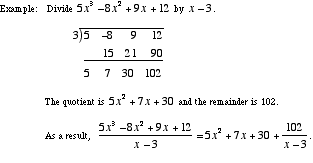index: click on a letter A B C D E F G H I J K L M N O P Q R S T U V W X Y Z A to Z index index: subject areas numbers & symbols sets, logic, proofs geometry algebra trigonometry advanced algebra & pre-calculus calculus advanced topics probability & statistics real world applications multimedia entrieswww.mathwords.com about mathwords website feedback

 Synthetic Division A shortcut for polynomial long division that can be used when dividing by an expression of the form x – c or x + c. Note: This allows an improper rational expression to be written as a sum of a polynomial and a proper rational expression.See also Synthetic substitution